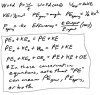﻿ Untitled 1

Work Energy Test:

Concepts and Skills:

• Formulas that you should memorize (if you want extra points):• Be able to define all of the items in the above formulas.  You won't actually have to define them, but you should know what everything means.
• Units and their abbreviations, symbols, etc.  (work, types of energy, power, efficiency)
• Know when each of the formulas can be applied, how it works, how (or if) its application may vary, and what units need to be used.
• Know how to work with, and convert between, different units for the same quantities.  For power, some units are horsepower and Watts.  For energy, some units are kiloWatt-hours, Watt-hours, Joules, calories, and food calories.  Any necessary conversion ratios will be provided.  You do not need to memorize them.
• Calculate work done by a varying force.
• Apply the various forms of the conservation of energy equation to a variety of situations.
• Derive equations by solving problems with variable names rather than concrete numbers.

Format:

• 13 Multiple choice questions
• 6 Problems, with 14 total parts
• Some relatively straightforward work and power problems.
• One problem relating to electrical energy
• One problem focusing on conservation of energy (no non-conservative work)
• One problem with non-conservative work
• A multi-part roller coaster problem